# Python | Linear Regression using sklearn

• Difficulty Level : Easy
• Last Updated : 23 Aug, 2022

Prerequisite: Linear Regression Linear Regression is a machine learning algorithm based on supervised learning. It performs a regression task. Regression models a target prediction value based on independent variables. It is mostly used for finding out the relationship between variables and forecasting. Different regression models differ based on – the kind of relationship between dependent and independent variables, they are considering and the number of independent variables being used. This article is going to demonstrate how to use the various Python libraries to implement linear regression on a given dataset. We will demonstrate a binary linear model as this will be easier to visualize. In this demonstration, the model will use Gradient Descent to learn. You can learn about it here.

Step 1: Importing all the required libraries

## Python3

 `import` `numpy as np``import` `pandas as pd``import` `seaborn as sns``import` `matplotlib.pyplot as plt``from` `sklearn ``import` `preprocessing, svm``from` `sklearn.model_selection ``import` `train_test_split``from` `sklearn.linear_model ``import` `LinearRegression`

Step 2: Reading the dataset You can download the dataset

## Python3

 `df ``=` `pd.read_csv(``'bottle.csv'``)``df_binary ``=` `df[[``'Salnty'``, ``'T_degC'``]]`` ` `# Taking only the selected two attributes from the dataset``df_binary.columns ``=` `[``'Sal'``, ``'Temp'``]``#display the first 5 rows``df_binary.head()`

Output: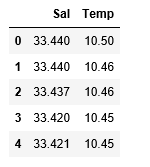Step 3: Exploring the data scatter

## Python3

 `#plotting the Scatter plot to check relationship between Sal and Temp``sns.lmplot(x ``=``"Sal"``, y ``=``"Temp"``, data ``=` `df_binary, order ``=` `2``, ci ``=` `None``)`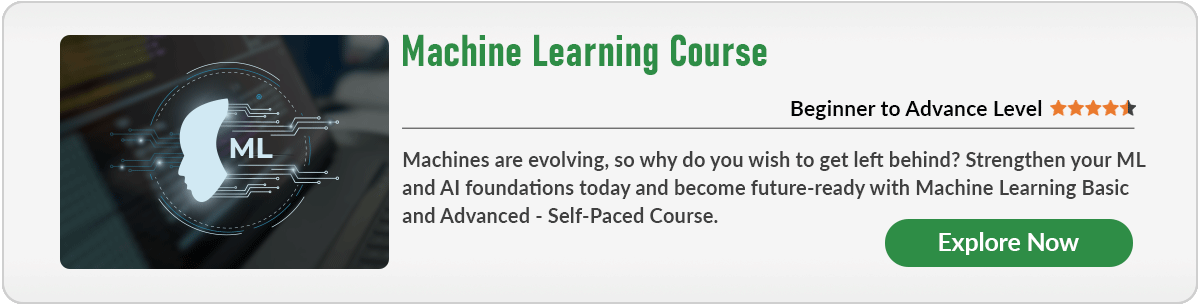Output: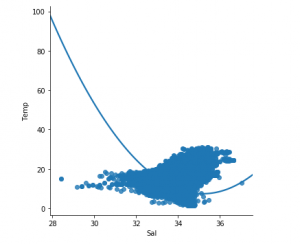Step 4: Data cleaning

## Python3

 `# Eliminating NaN or missing input numbers``df_binary.fillna(method ``=``'ffill'``, inplace ``=` `True``)`

Step 5: Training our model

## Python3

 `X ``=` `np.array(df_binary[``'Sal'``]).reshape(``-``1``, ``1``)``y ``=` `np.array(df_binary[``'Temp'``]).reshape(``-``1``, ``1``)`` ` `# Separating the data into independent and dependent variables``# Converting each dataframe into a numpy array ``# since each dataframe contains only one column``df_binary.dropna(inplace ``=` `True``)`` ` `# Dropping any rows with Nan values``X_train, X_test, y_train, y_test ``=` `train_test_split(X, y, test_size ``=` `0.25``)`` ` `# Splitting the data into training and testing data``regr ``=` `LinearRegression()`` ` `regr.fit(X_train, y_train)``print``(regr.score(X_test, y_test))`

Output: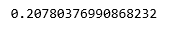Step 6: Exploring our results

## Python3

 `y_pred ``=` `regr.predict(X_test)``plt.scatter(X_test, y_test, color ``=``'b'``)``plt.plot(X_test, y_pred, color ``=``'k'``)`` ` `plt.show()``# Data scatter of predicted values`

Output: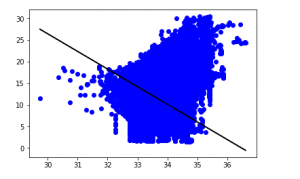The low accuracy score of our model suggests that our regressive model has not fit very well with the existing data. This suggests that our data is not suitable for linear regression. But sometimes, a dataset may accept a linear regressor if we consider only a part of it. Let us check for that possibility.

Step 7: Working with a smaller dataset

## Python3

 `df_binary500 ``=` `df_binary[:][:``500``]``   ` `# Selecting the 1st 500 rows of the data``sns.lmplot(x ``=``"Sal"``, y ``=``"Temp"``, data ``=` `df_binary500,``                               ``order ``=` `2``, ci ``=` `None``)`

Output: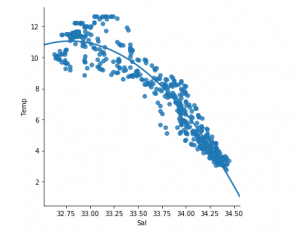We can already see that the first 500 rows follow a linear model. Continuing with the same steps as before.

## Python3

 `df_binary500.fillna(method ``=``'ffill'``, inplace ``=` `True``)`` ` `X ``=` `np.array(df_binary500[``'Sal'``]).reshape(``-``1``, ``1``)``y ``=` `np.array(df_binary500[``'Temp'``]).reshape(``-``1``, ``1``)`` ` `df_binary500.dropna(inplace ``=` `True``)``X_train, X_test, y_train, y_test ``=` `train_test_split(X, y, test_size ``=` `0.25``)`` ` `regr ``=` `LinearRegression()``regr.fit(X_train, y_train)``print``(regr.score(X_test, y_test))`

Output: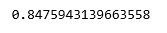## Python3

 `y_pred ``=` `regr.predict(X_test)``plt.scatter(X_test, y_test, color ``=``'b'``)``plt.plot(X_test, y_pred, color ``=``'k'``)`` ` `plt.show()`

Output: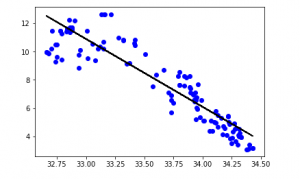Step 8: Evaluation Metrics For Regression

At last, we check the performance of the Linear Regression model with help of evaluation metrics. For Regression algorithms we widely use mean_absolute_error, and mean_squared_error metrics to check the model performance.

## Python3

 `from` `sklearn.metrics ``import` `mean_absolute_error,mean_squared_error`` ` `mae ``=` `mean_absolute_error(y_true``=``y_test,y_pred``=``y_pred)``#squared True returns MSE value, False returns RMSE value.``mse ``=` `mean_squared_error(y_true``=``y_test,y_pred``=``y_pred) ``#default=True``rmse ``=` `mean_squared_error(y_true``=``y_test,y_pred``=``y_pred,squared``=``False``)`` ` `print``(``"MAE:"``,mae)``print``(``"MSE:"``,mse)``print``(``"RMSE:"``,rmse)`

Output:

```MAE: 0.7927322046360309
MSE: 1.0251137190180517
RMSE: 1.0124789968281078```

My Personal Notes arrow_drop_up